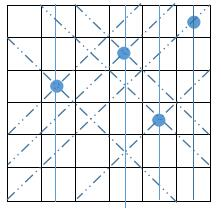# N-Queens Problem

## Overview

The eight queens problem is a question with chess as the background: how to place eight queens on an 8×8 chess board so that no queen can directly eat other queens? In order to achieve this purpose, any two queens are not in the same horizontal, vertical or diagonal. The eight queen problem can be extended to the more general N-Queens Problem.

## Introduction

### initialization

Upperlim value is n-bit binary 1.

### Recursive Function

The function has three parameters:

• row : row
• ld : Left diagonal
• rd : Right diagonal

Use these three parameters to determine whether a queen can be placed in a certain position.

### Diagram

Here is a step-by-step illustration of the Six Queens question.The first round of search is completed, there is a kind of thought is the depth first search, is to find a solution and then press forward to the enemy’s capital to find second solution, because the eight queens problem belongs to the scope of the graph, so the idea of graph theory can be found in this problem.

Share